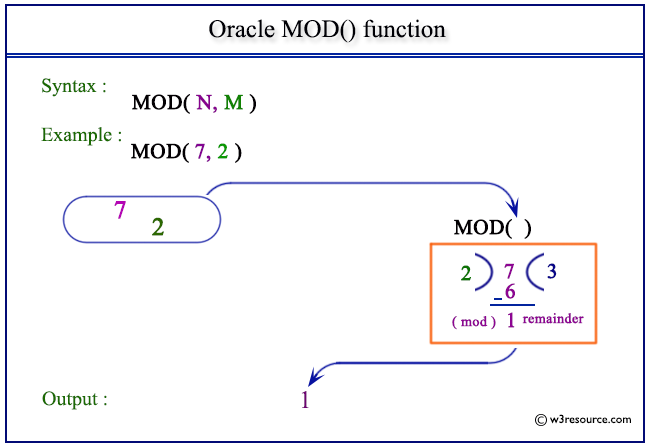# Oracle MOD() function

## Description

The Oracle MOD() is used to return the remainder of a dividend divided by a divisor. This function also works on fractional values and returns the exact remainder. The function returns dividend when the value of divisor is 0.
The function takes any numeric or nonnumeric data type (can be implicitly converted to a numeric data type) as an argument.
If the argument is BINARY_FLOAT, then the function returns BINARY_DOUBLE. Otherwise, the function returns the same numeric data type as the argument

Syntax:

`MOD(N,M)`

Arguments:

Name Description
N Dividend.
M Divisor.

Pictorial Presentation of MOD() functionExample-1:

The statement below returns the remainder of17 divided by 2.

``````SELECT MOD(7,2) FROM dual;
```
```

Here is the result.

```  MOD(7,2)
----------
1
```

Example-2:

The statement below returns the remainder of 7 divided by -2.

``````SELECT MOD(7,-2) FROM dual;
```
```

Here is the result.

```  MOD(7,-2)
----------
1
```

Example-3:

The statement below returns the remainder of -7 divided by 2.

``````SELECT MOD(-7, 2);
```
```

Here is the result.

``` MOD(-7,2)
----------
-1
```

Example-4:

The statement below returns the remainder of -7 divided by -2.

``````SELECT MOD(-7, -2);
```
```

Here is the result.

``` MOD(-7,-2)
----------
-1
```

Previous: LOG
Next: NANVL

﻿